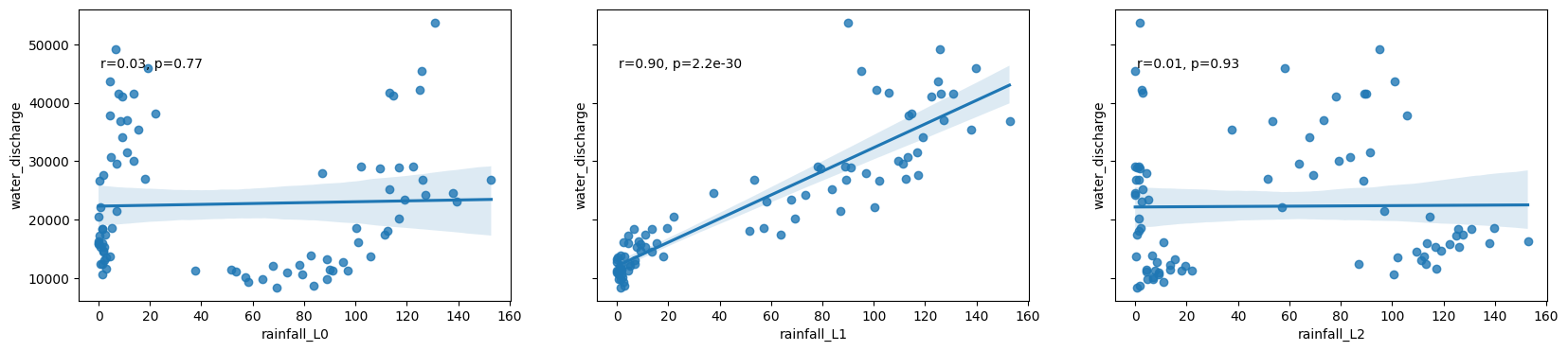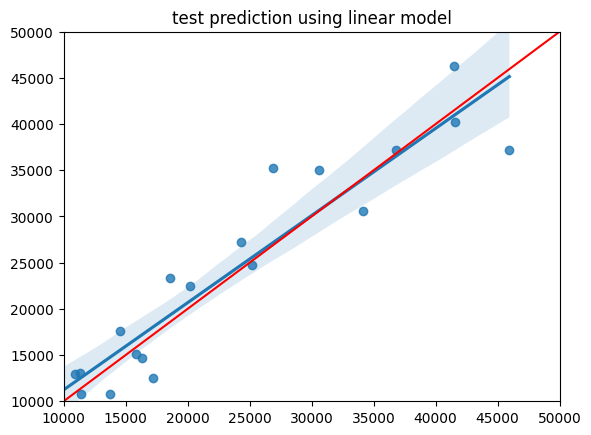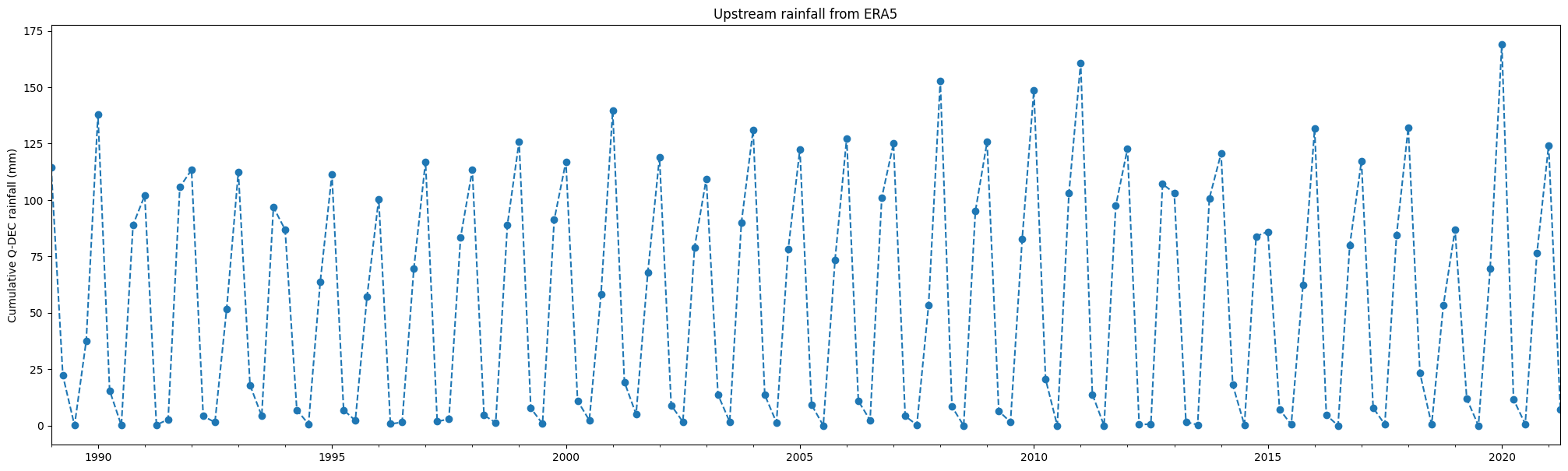# Modelling discharge at Mohembo using uppercatchment rainfall¶

• Products used: ERA5

## Description¶

The discharge data at Mohembo becomes much more sparse during the era of good quality satellite observations, making it unreliable for comparing discharge with surface water extent. This notebook will try to model the discharge at Mohembo using the upstream rainfall extracted from ERA5 in a previous notebook

## Getting started¶

To run this analysis, run all the cells in the notebook, starting with the “Load packages” cell.

Import Python packages that are used for the analysis.

:

%matplotlib inline
import warnings
import datetime as dt
import numpy as np
import pandas as pd
import seaborn as sns
from scipy import stats
import matplotlib.pyplot as plt
from sklearn.metrics import mean_absolute_error
from sklearn.linear_model import LinearRegression
from sklearn.ensemble import RandomForestRegressor
from sklearn.model_selection import train_test_split

from deafrica_tools.spatial import xr_rasterize

/env/lib/python3.8/site-packages/geopandas/_compat.py:106: UserWarning: The Shapely GEOS version (3.8.0-CAPI-1.13.1 ) is incompatible with the GEOS version PyGEOS was compiled with (3.9.1-CAPI-1.14.2). Conversions between both will be slow.
warnings.warn(

:

warnings.filterwarnings("ignore")


## Analysis Parameters¶

:

upstream_rainfall_1989_2009 = 'results/upstream_rainfall_daily_1989-01_to_2009-12.csv'

upstream_rainfall_2010_2021 = 'results/upstream_rainfall_daily_2010-01_to_2021-05-26.csv'

freq='Q-DEC'

max_lags = 3

sklearn_model = RandomForestRegressor


## Retrieve historical rainfall data over all areas of interest¶

This data has already been retrieved from ERA5 so we can simply pull in the csv on disk

:

upstream_rainfall_1989_2009 = pd.read_csv(upstream_rainfall_1989_2009, index_col='time',parse_dates=True)
rain = pd.concat([upstream_rainfall_1989_2009, upstream_rainfall_2010_2021])


No need to re-run this since the data has been saved in the results/ folder

:

# # Original shapefile from https://data.apps.fao.org/map/catalog/srv/api/records/57bb1c95-2f00-4def-886f
# vector_file = 'data/OB_FWR_Hydrography_Okavango_Subasins_polygon.geojson'

# # define time period of interest
# time_range = '1989-01', '2009-12'

# # Original shapefile from https://data.apps.fao.org/map/catalog/srv/api/records/57bb1c95-2f00-4def-886f-caee3d756da9

# # upstream include Cuito and Cubango subbasins
# upstream = basin[basin.Subbasin.isin(['Cuito', 'Cubango'])]
# print(upstream)

# # get historical rainfall for upstream and delta
# bounds = upstream.total_bounds
# lat = bounds, bounds
# lon = bounds, bounds

# var = 'precipitation_amount_1hour_Accumulation'
# precip = load_era5(var, lat, lon, time_range, reduce_func=np.sum, resample='1D').compute()

# # fix inconsistency in axis names
# precip = precip.rename({'lat':'latitude', 'lon':'longitude'})

# upstream_raster = xr_rasterize(upstream, precip, x_dim='longitude', y_dim='latitude')

# upstream_rainfall = precip[var].where(upstream_raster).sum(['latitude','longitude'])

# upstream_rainfall.to_dataframe().drop('spatial_ref',axis=1).rename({'precipitation_amount_1hour_Accumulation':'cumulative daily rainfall (mm)'},axis=1).to_csv(f'results/upstream_rainfall_daily_{time_range}_to_{time_range}.csv')


## Import discharge data¶

:

discharge = 'data/mohembo_daily_water_discharge_data.csv'
dis['date'] = pd.to_datetime(dis['date'], dayfirst=True)
dis = dis.set_index('date')


### Match discharge with rainfall¶

:

dis = dis.loc[(dis.index >= rain.index)]

:

df = rain.join(dis, how='outer')
df.tail()

:

cumulative daily rainfall (mm) water_discharge
2021-05-22 0.0 201.356
2021-05-23 0.0 202.850
2021-05-24 0.0 200.148
2021-05-25 0.0 192.258
2021-05-26 0.0 193.073

### Resample seasonal or monthly cumulative totals¶

By intergrating rainfall over a longer time-period (months to seasons) we can better correlate cumulative upstream rainfall with discharge at Mohembo.

:

#total rainfall per month
df = df.resample(freq).sum()

:

cumulative daily rainfall (mm) water_discharge
1989-03-31 114.574218 41274.290
1989-06-30 22.230042 38089.340
1989-09-30 0.119446 20520.900
1989-12-31 37.682495 11343.479
1990-03-31 137.858338 24604.860

Split dataset at 2010. That way we can build a model on the historical, complete dataset, and then predict on the incomplete record from 2010 onwards

:

df_2010 = df.loc[(df.index >= pd.to_datetime('2010-01-01'))]
df_1989 = df.loc[(df.index < pd.to_datetime('2010-01-01'))]
df_1989.tail()

:

cumulative daily rainfall (mm) water_discharge
2008-12-31 95.044922 12850.7990
2009-03-31 125.729308 45340.2135
2009-06-30 6.483582 49117.4010
2009-09-30 1.598633 18477.3465
2009-12-31 82.677734 13901.3405

## Explore correlations with lags in rainfall¶

:

def crosscorr(datay, datax, lag=0):
"""
Lag-N cross correlation.

lag : int, default 0
datax, datay : pandas.Series objects of equal length

"""
return datay.corr(datax.shift(lag))

:

# calculate cross correlation for each lag period
xcov = [crosscorr(df_1989['water_discharge'], df_1989['cumulative daily rainfall (mm)'], lag=i) for i in range(max_lags)]

# Scatter plots of the relationship between rainfall and discharge at each lag
fig, ax = plt.subplots(1,max_lags, figsize=(20,4), sharey=True)
for lag in range(max_lags):
df_1989['rainfall_L'+str(lag)] = df_1989['cumulative daily rainfall (mm)'].shift(lag)
sns.regplot(x='rainfall_L'+str(lag), y='water_discharge', data=df_1989, ax=ax[lag])
r, p = stats.pearsonr(df_1989['rainfall_L'+str(lag)][lag:], df_1989['water_discharge'][lag:])
ax[lag].text(.05, .8, 'r={:.2f}, p={:.2g}'.format(r, p), transform=ax[lag].transAxes)## Regression modelling¶

Model the relationship between rainfall and discharge using the lag that corresponds with the highest correlation

Conduct a test/train split first to get an idea of the general accuracy of this approach

:

max_value = max(xcov)
best_lag = xcov.index(max_value)
print("Best lag:",best_lag)

Best lag: 1

:

#convert data into a format that scikit learn likes
X = df_1989['rainfall_L'+str(best_lag)][best_lag:].values.reshape(-1,1)
y = df_1989['water_discharge'][best_lag:].values.reshape(-1,1)

#conduct a train test split
X_train,X_test, y_train, y_test = train_test_split(X, y, test_size=0.25, random_state=1)

#model the relationship using just the training data, then predict on test data
model = sklearn_model()
model.fit(X_train,y_train)
y_pred = model.predict(X_test)

print('MAE: ',mean_absolute_error(y_test,y_pred))

#plot result of test data
sns.regplot(x=y_test, y=y_pred)
plt.xlim(10000, 50000)
plt.ylim(10000, 50000)
plt.plot([10000, 50000], [10000, 50000], 'k-', color = 'r')
plt.title('test prediction using linear model');

MAE:  3356.3316369380896## Predict Discharge at Mohembo¶

Grab the rainfall from the period we want to predict

:

fc_rainfall=df['cumulative daily rainfall (mm)'].shift(best_lag)
X_fc = fc_rainfall[best_lag:].values.reshape(-1,1)


Make a prediction using rainfall data

:

# model = LinearRegression()
model = sklearn_model()
model.fit(X,y) #train 1989-2010
fc = model.predict(X_fc) # predict on all data


## Plot the observed vs predicted discharge as a time series¶

:

df_predicted = df[best_lag:]
df_predicted['predicted_discharge'] = fc.reshape(-1)

:

cumulative daily rainfall (mm) water_discharge predicted_discharge
1989-06-30 22.230042 38089.34 36960.159500
1989-09-30 0.119446 20520.90 19621.901129

### Plot rainfall¶

:

df['cumulative daily rainfall (mm)'].plot(linestyle='dashed', marker='o',figsize=(25,7))
plt.title('Upstream rainfall from ERA5')
plt.ylabel('Cumulative '+freq+' rainfall (mm)')
plt.xlabel('');:

data = 'results/okavango_all_datasets.csv'


### Save result to disk¶

:

df_predicted.rename({'cumulative daily rainfall (mm)':'upstream_rainfall'}, axis=1).to_csv('results/modelled_discharge_'+freq+'.csv')


Contact: If you need assistance, please post a question on the Open Data Cube Slack channel or on the GIS Stack Exchange using the open-data-cube tag (you can view previously asked questions here). If you would like to report an issue with this notebook, you can file one on Github.

Last Tested:

:

from datetime import datetime
datetime.today().strftime('%Y-%m-%d')

:

'2021-09-14'